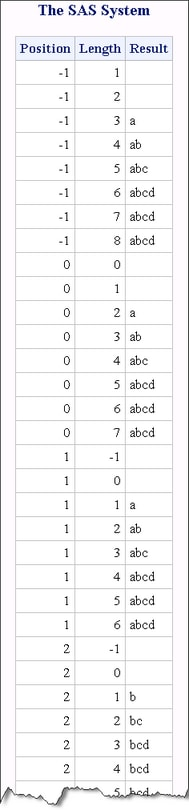# SUBSTRN Function

Returns a substring, allowing a result with a length of zero.

 Category: Character Restriction: I18N Level 1 functions should be avoided, if possible, if you are using a non-English language. The I18N Level 1 functions might not work correctly with Double Byte Character Set (DBCS) or Multi-Byte Character Set (MBCS) encodings under certain circumstances. Tip: has the same functionality.

## Syntax

SUBSTRN(string, position <, length>)

### Required Arguments

#### string

specifies a character or numeric constant, variable, or expression.

If string is numeric, then it is converted to a character value that uses the BEST32. format. Leading and trailing blanks are removed, and no message is sent to the SAS log.

#### position

is an integer that specifies the position of the first character in the substring.

### Optional Argument

#### length

is an integer that specifies the length of the substring. If you do not specify length, the SUBSTRN function returns the substring that extends from the position that you specify to the end of the string.

## Details

### Length of Returned Variable

In a DATA step, if the SUBSTRN function returns a value to a variable that has not previously been assigned a length, then that variable is given the length of the first argument.

### The Basics

The following information applies to the SUBSTRN function:
• The SUBSTRN function returns a string with a length of zero if either position or length has a missing value.
• If the position that you specify is non-positive, the result is truncated at the beginning, so that the first character of the result is the first character of the string. The length of the result is reduced accordingly.
• If the length that you specify extends beyond the end of the string, the result is truncated at the end, so that the last character of the result is the last character of the string.

### Using the SUBSTRN Function in a Macro

If you call SUBSTRN by using the %SYSFUNC macro, then the macro processor resolves the first argument (string) to determine whether the argument is character or numeric. If you do not want the first argument to be evaluated as a macro expression, use one of the macro-quoting functions in the first argument.

## Comparisons

The following table lists comparisons between the SUBSTRN and the SUBSTR functions:
Condition
Function
Result
the value of position is nonpositive
SUBSTRN
returns a result beginning at the first character of the string.
the value of position is nonpositive
SUBSTR
• writes a note to the log stating that the second argument is invalid.
• sets _ERROR_ =1.
• returns the substring that extends from the position that you specified to the end of the string.
the value of length is nonpositive
SUBSTRN
returns a result with a length of zero.
the value of length is nonpositive
SUBSTR
• writes a note to the log stating that the third argument is invalid.
• sets _ERROR_ =1.
• returns the substring that extends from the position that you specified to the end of the string.
the substring that you specify extends past the end of the string
SUBSTRN
truncates the result.
the substring that you specify extends past the end of the string
SUBSTR
• writes a note to the log stating that the third argument is invalid.
• sets _ERROR_=1.
• returns the substring that extends from the position that you specified to the end of the string.

## Examples

### Example 1: Manipulating Strings with the SUBSTRN Function

The following example shows how to manipulate strings with the SUBSTRN function.
```data test;
retain string "abcd";
drop string;
do Position = -1 to 6;
do Length = max(-1,-position) to 7-position;
Result = substrn(string, position, length);
output;
end;
end;
datalines;
abcd
;
proc print noobs data=test;
run;```
Output from the SUBSTRN Function### Example 2: Comparison between the SUBSTR and SUBSTRN Functions

The following example compares the results of using the SUBSTR function and the SUBSTRN function when the first argument is numeric.
```data _null_;
substr_result = "*" || substr(1234.5678,2,6) || "*";
put substr_result=;
substrn_result = "*" || substrn(1234.5678,2,6)  || "*";
put substrn_result=;
run;```
Results from the SUBSTR and SUBSTRN Functions
```substr_result=*  1234*
substrn_result=*234.56*```